# Functions Inverse Relation Let R be a relation

• Slides: 16FunctionsInverse Relation • Let R be a relation from X to Y. • R-1 = {y. R-1 x | x. Ry } • Examples of R & R-1: • x < y & y > x. • x | y & y is an integral multiple of x.Functions • • R(a) = { b | a. Rb } is the image of a under R. If R is an equivalence relation, then R(a) = [a]. Let A & B be nonempty sets. A function f : A B is a relation from A to B such that: • a A, b B, a f b, denoted f(a) = b Every element of A has at least 1 image. • [ f(x) = y f(x) = z ] y = z. Every element of A has at most 1 image.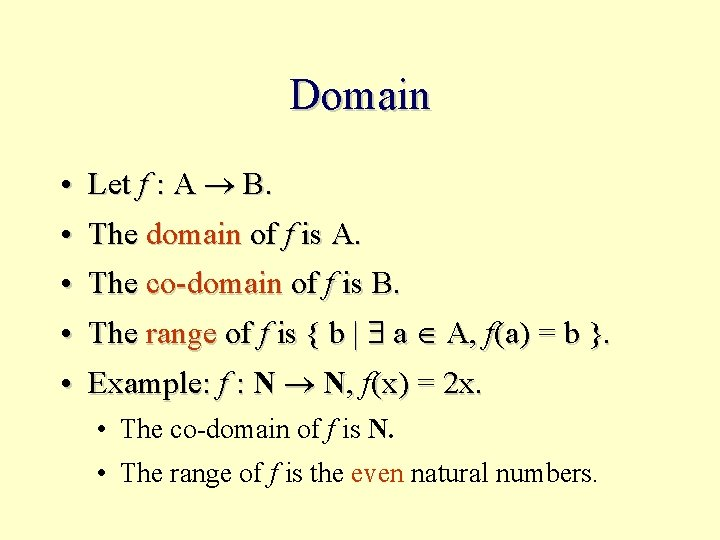Domain • Let f : A B. • The domain of f is A. • The co-domain of f is B. • The range of f is { b | a A, f(a) = b }. • Example: f : N N, f(x) = 2 x. • The co-domain of f is N. • The range of f is the even natural numbers.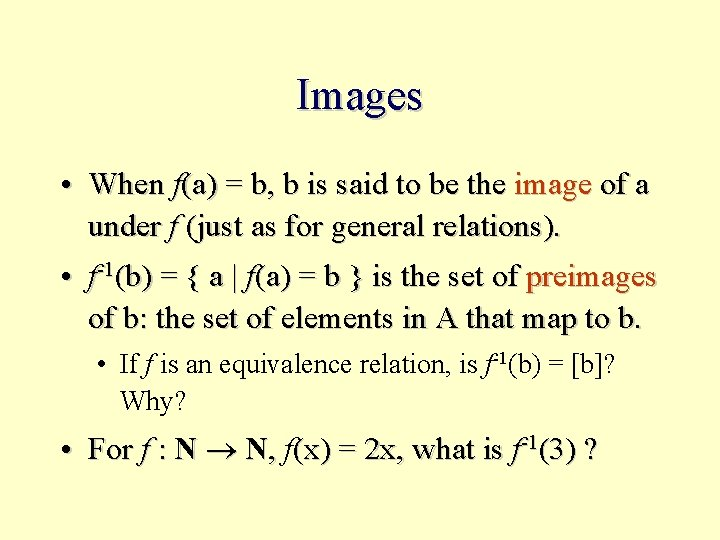Images • When f(a) = b, b is said to be the image of a under f (just as for general relations). • f-1(b) = { a | f(a) = b } is the set of preimages of b: the set of elements in A that map to b. • If f is an equivalence relation, is f-1(b) = [b]? Why? • For f : N N, f(x) = 2 x, what is f-1(3) ?Visualizing Functions • Visualize functions via the vertical line test. • A relation that violates rule 1: every element has an image • A relation that violates rule 2: every element’s image is unique. • A graph that is discontinuous. • A graph where the co-domain the range.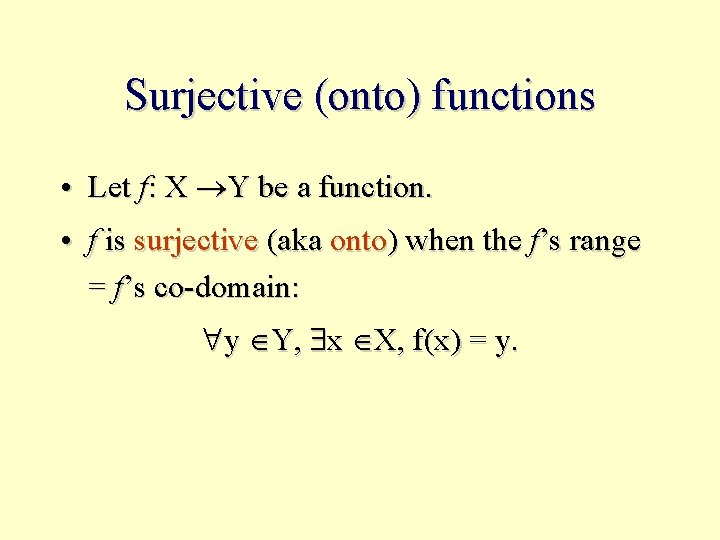Surjective (onto) functions • Let f: X Y be a function. • f is surjective (aka onto) when the f’s range = f’s co-domain: y Y, x X, f(x) = y.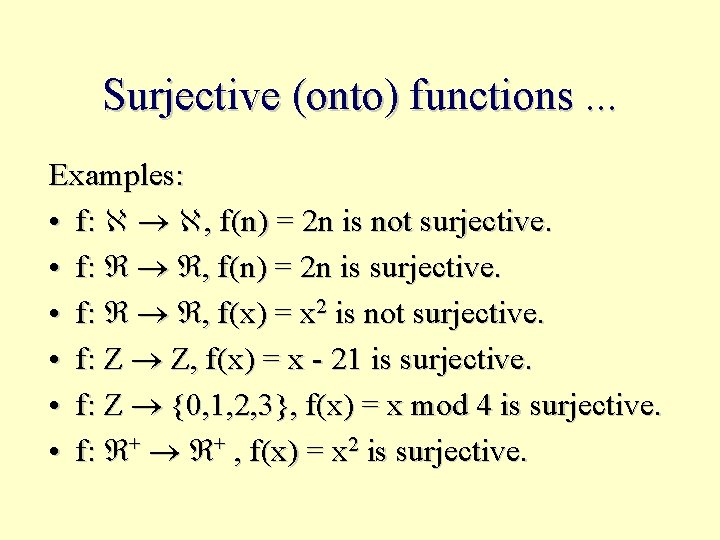Surjective (onto) functions. . . Examples: • f: , f(n) = 2 n is not surjective. • f: , f(n) = 2 n is surjective. • f: , f(x) = x 2 is not surjective. • f: Z Z, f(x) = x - 21 is surjective. • f: Z {0, 1, 2, 3}, f(x) = x mod 4 is surjective. • f: + + , f(x) = x 2 is surjective.Injective Functions • Function f is injective when x y f(x) f(y). • Examples: • f: Z Z, f(x) = x 2 (injective? ) • f: Z Z, f(x) = 2 x (injective? ) • f: Z {0, 1, 2, 3}, f(x) = x mod 4 (injective? )Bijective Functions • A function is bijective when it is surjective and injective. • A bijective function also is known as a 1 -to 1 correspondence. • Examples: • f: Z Z, f(x) = x + 3 (bijective? ) • f: , f(x) = 2 x (bijective? )Invertible Functions • Let f: X Y be a function. • Let f-1: Y X be the inverse relation: f-1 = {(y, x) | f(x) = y}. • Theorem: f-1 is a function if and only if f is a bijection.Proof ( ): If f-1 is a function then f is bijective. • f-1 is a function: • y Y, x X, f-1 (y) = x. • This means f is surjective. (Illustrate) • [f-1 (y) = x f-1 (y) = z ] x = z • This means f is injective. (Illustrate) • Therefore, f is bijective.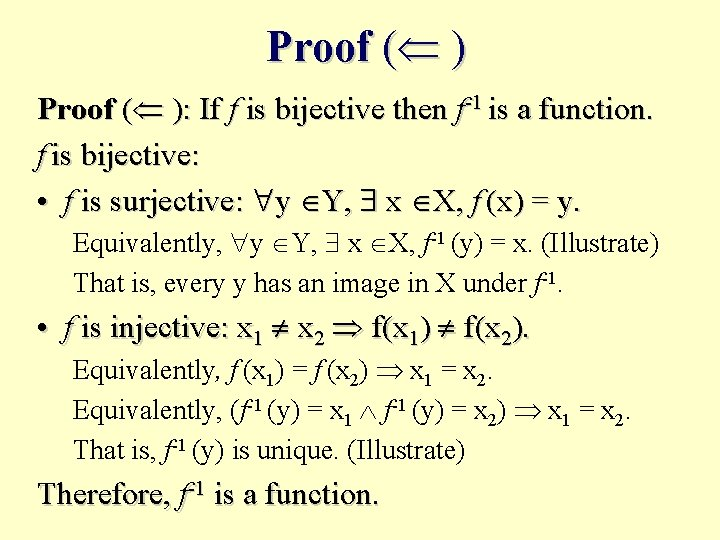Proof ( ): If f is bijective then f-1 is a function. f is bijective: • f is surjective: y Y, x X, f (x) = y. Equivalently, y Y, x X, f-1 (y) = x. (Illustrate) That is, every y has an image in X under f-1. • f is injective: x 1 x 2 f(x 1) f(x 2). Equivalently, f (x 1) = f (x 2) x 1 = x 2. Equivalently, (f-1 (y) = x 1 f-1 (y) = x 2) x 1 = x 2. That is, f-1 (y) is unique. (Illustrate) Therefore, f-1 is a function.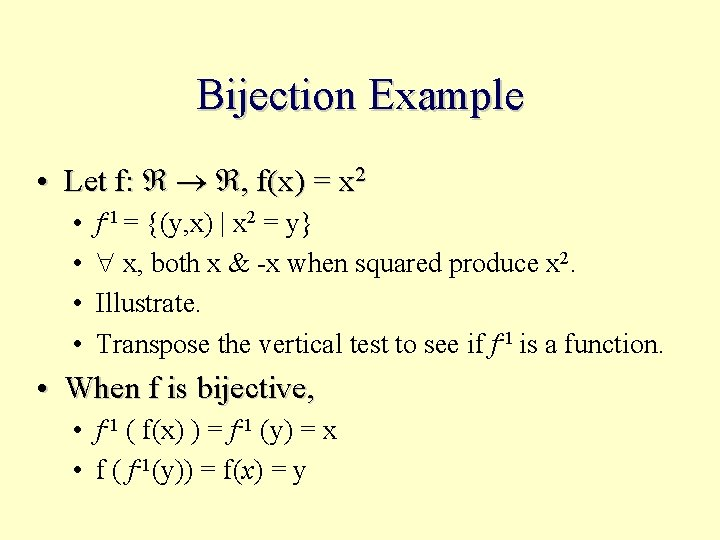Bijection Example • Let f: , f(x) = x 2 • • f-1 = {(y, x) | x 2 = y} x, both x & -x when squared produce x 2. Illustrate. Transpose the vertical test to see if f-1 is a function. • When f is bijective, • f-1 ( f(x) ) = f-1 (y) = x • f ( f-1(y)) = f(x) = y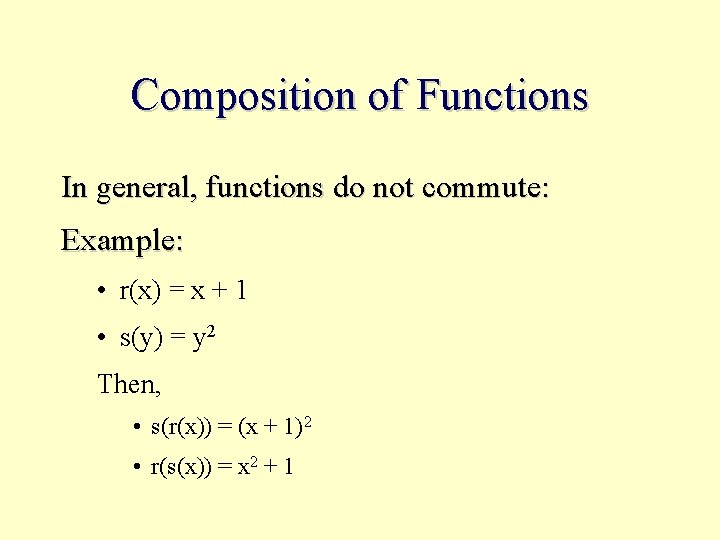Composition of Functions In general, functions do not commute: Example: • r(x) = x + 1 • s(y) = y 2 Then, • s(r(x)) = (x + 1)2 • r(s(x)) = x 2 + 1Characters • • •